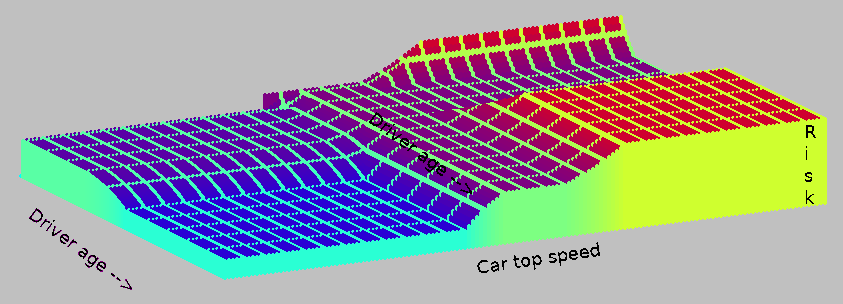# Fuzzy Logic# What is fuzzy logic?

In many human situations there are parameters that can be measured and are associated with choice of an outcome, but there is no definable 'equation'. Rather we can gather data from previous cases and use these to classify new cases.

Suppose a company business is based on providing car insurance. Driver ages are 18 to 120, which we could divide into ranges -

driver.is.young ( 18, 25) - driver.is.middle ( 25, 70) - driver.is.old ( 70+)

Similarly, car top speed is relevant.

Top speed of the car is likely to be relevant- car top speed ( <120), ( <140) ( <170)

Combinations of these amount to 'rules'. But the boundaries are not sharp, but graduated. This is the 'fuzziness'

If driver is young OR old, and car is fast, then risk is high

If driver is middle-aged, OR car is average-speed, then risk is middle

If car is slow then risk is low except for young drivers

## Code below is largely my take on code from bluatigro on the forum

```'   *** Fuzzy logic example- bluatigro+jhf  ****

WindowWidth  = 1000
WindowHeight =  900

nomainwin

open "Fuzzy insurance.inc" for graphics_nf_nsb as #m
#m "trapclose quit"
#m "fill lightgray"
#m "size 4"

for age =18 to 120
driver.is.young\$  = fuz\$(   0,   0,  18,  25)
driver.is.middle\$ = fuz\$(  18,  25,  50,  70)
driver.is.old\$    = fuz\$(  50,  70, 120, 120)

for vel =0 to 300 step 2
car.is.slow\$      = fuz\$(   0,   0, 120, 140)
car.is.average\$   = fuz\$( 120, 140, 170, 200)
car.is.fast\$      = fuz\$( 170, 200, 400, 400)

driverYoung       = fuzz( age, driver.is.young\$)
driverMiddle      = fuzz( age, driver.is.middle\$)
driverOld         = fuzz( age, driver.is.old\$)

carSlow           = fuzz( vel,  car.is.slow\$)
carAverage        = fuzz( vel,  car.is.average\$)
carFast           = fuzz( vel,  car.is.fast\$)

''if driver is young OR old, and car is fast, then risk is high
riskHigh          = min( carFast, max( driverYoung, driverOld))

''if driver is middle-aged, OR car is average-speed, then risk is middle
riskMiddle        = max( driverMiddle, carAverage)

''if car is slow then risk is low except for young drivers
riskLow           = min( carSlow, 1 -driverYoung)

''defuzzyfication
pay.is.low\$      =fuz\$(  0,  1, 20,  40)
pay.is.middle\$   =fuz\$( 20, 40, 60,  80)
pay.is.high\$     =fuz\$( 60, 80, 99, 100)

ml               =mass(  riskLow,    pay.is.low\$)
mm               =mass(  riskMiddle, pay.is.middle\$)
mh               =mass(  riskHigh,   pay.is.high\$)

pl               =point( pay.is.low\$)
pm               =point( pay.is.middle\$)
ph               =point( pay.is.high\$)

pay = ( ml *pl +mm *pm +mh *ph) /( ml +mm+ mh +1E-10)

call plot vel, age, pay

scan
next vel
next age

#m "flush"

wait

sub plot x, y, z
r = z * 2.5
if not( x mod 10) or not( y mod 10) then g = 255
b = 255 - r 'z * 2.5
#m "color "; r ;" "; g; " "; b
#m "down"
#m "line "; 100 +2 *y +2 *x; " "; 400 +y -x /4; " "; 100+ 2 *y +2*x; " "; 400 +y -x /4 -z
#m "up"
end sub

function fuz\$( a, b, c, d)  ''construct a fuzzy-object\$
fuz\$ =a; " "; b; " "; c; " "; d
end function

function fuzz( x, f\$)  ''what is membervalue of x in fuzzy-object f\$
a    =val( word\$( f\$, 1))
b    =val( word\$( f\$, 2))
c    =val( word\$( f\$, 3))
d    =val( word\$( f\$, 4))
uit  =0
if ( b <=x) and ( x <=c)  then uit =1
if ( a

```